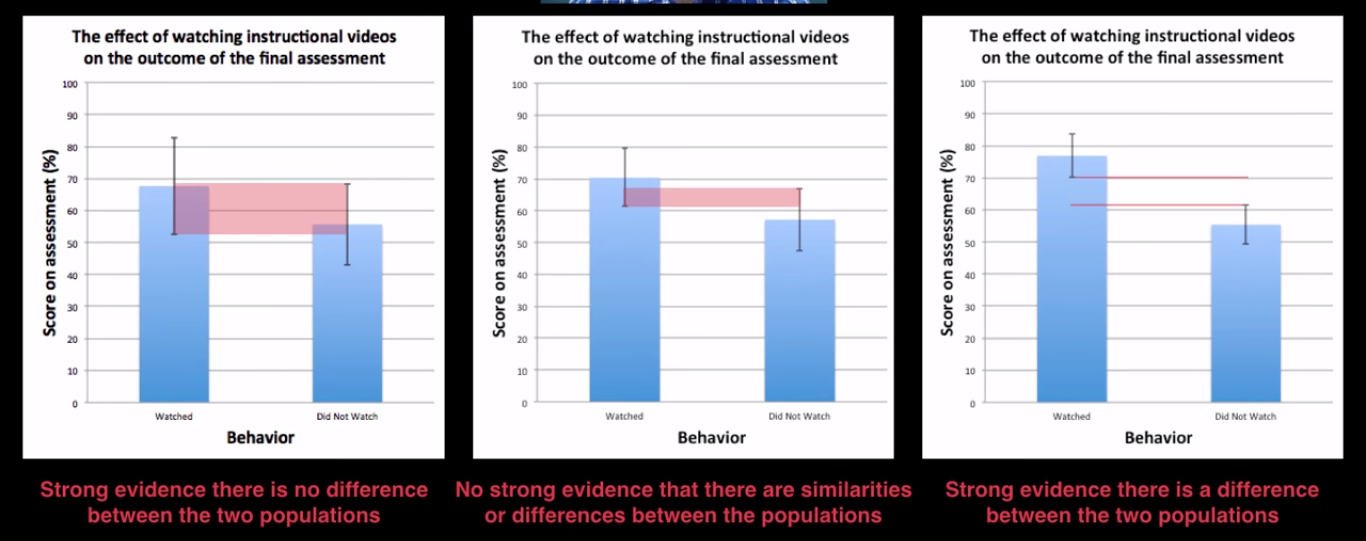This is my personal cheatsheet for statistics. It is far from being a complete introduction. $\def\mean#1{\bar #1} \DeclareMathOperator{\EV}{\mathbb{E}}$

Intro to Research Methods

Construcs

Something hard to measure, that you’ll have to define how to measure.

Operational Definition

It’s how you will measure a given construct.

Parameters vs Statistics

Parameters refer to the whole population. Statistics refer to the sample (part of the population).

Relationships vs Causation

Relationships can be shown by observational studies (eg. by seeing a point distribution). Causation, however, has to be found through controlled experiments.

Measures of central tendency

Mode

The value at which frequency is highest.

The mode doesn’t really represent the data well because:

• It’s not affected by all scores in the dataset;
• It changes radically depending on the sample;
• We can’t really describe the mode by an equation.

Median

The value in the middle of the distribution.

(If there are an even number of elements, the median will be the average of the two in the middle.)

The median is more robust to outliers than the mean. It is therefore the best measure of central tendency when dealing with highly skewed distributions.

Mean (expected value)

The weighted average, or expected value.

• Population mean: $\mu$
• Sample mean: $\mean{x}$ (can be used to estimate $\mu$)
• The mean is affected by outliers.The mean is always pulled towards the longest tail of the distribution, in relation to the median.

Variability of data

Range

Range = MaxValue - MinValue

Interquartile Range (IQR)

IQR is the distance between the 25% and the 75% higher measure of the data.

IQR = Q3 - Q1

Problem with Range and IQR: neither takes all data into account.

Outlier

An observation point that is distant from other observations.

Values below (Q1 - 1.5*IQR) or above (Q3 + 1.5*IQR) are usually considered outliers.Outliers are represented as dots in a boxplot.

Estimators, Bias and Variance

(from a machine learning point of view)

Point Estimation

Point estimation is the attempt to provide the single “best” prediction of some quantity of interest. A point estimator or statistic is any function of the data:

The definition does not require that $g$ return a value that is close to the true $\boldsymbol{\theta}$ or even that the range of $g$ is the same as the set of allowable values of $\boldsymbol{\theta}$.

Bias

Bias measures the expected deviation from the true value of the function or parameter. The bias of an estimator is defined as:

where the expectation is over the data (seen as samples from a random variable).

Variance and Standard Deviation

Variance provides a measure of the deviation from the expected estimator value that any particular sampling of the data is likely to cause.

We call deviation a measure of difference between the observed value of a variable and some other value, often that variable’s mean. We calculate the variance by taking the mean of squared deviations:

The standard deviation is simply the root square of the variance. One interesting characteristic of the standard deviation is the 68–95–99.7 rule.

Bessel’s Correction (unbiased estimator of the population variance)

You use $(n - 1)$ instead of $(n)$ to calculate the standard deviation when estimating the standard deviation for the population from a sample.

Using the sample standard deviation (s) corrects the bias in the estimation of the population variance. It also partially corrects the bias in the estimation of the population standard deviation. However, the correction often increases the mean squared error in these estimations.

This short video tries to bring a bit more intuition on why to use $(n - 1)$ instead of $(n)$.

Standard Error of the Mean (SEM)

The SEM quantifies the precision of the mean, it is a measure of how far your sample mean is likely to be from the true mean of the population. But how to calculate it without doing lots of experiments and finding many different values for the mean? We can use the estimation:

Proof of the formula below (from here), the first step is allowed because the $X_i$ are independently sampled, so the variance of the sum is just the sum of the variances.

We can use the SEM to interpret different populations and see if there is evidence that they are differences or similarities between them:How the standard error of the mean helps us interpret populations, from this YouTube video.

The Bias vs Variance Tradeoff

How to choose between two estimators: one with more bias and another with more variance? The most common way to negotiate this trade-off is to use cross-validation. Alternatively, we can also compare the mean squared error (MSE) of the estimates:

The relationship between bias and variance is tightly linked to the machine learning concepts of capacity, underfitting and overfitting.